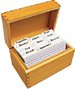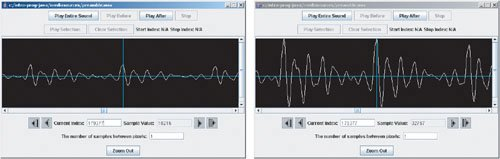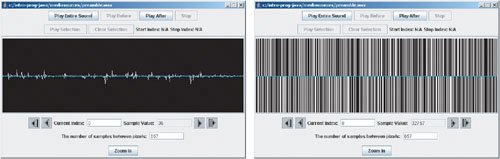# Section 8.4. Normalizing Sounds

[Page 280 (continued)]

### 8.4. Normalizing Sounds

If you think about it, it seems strange that the last two methods work! We can just multiply these numbers representing a soundand the sound seems (essentially) the same to our ears just louder? The way we experience a sound depends less on the specific numbers than on the relationship between them. Remember that the overall shape of the sound waveform is dependent on many samples. In general, if we multiply all the samples by the same multiplier, we only effect our sense of volume (intensity), not the sound itself. (We'll work to change the sound itself in future sections.)

A common operation that people want to do with sounds is to make them as LOUD AS POSSIBLE. That's called normalizing. It's not really hard to do, but it takes more lines of code than we've used previously and a few more variables, but we can do it. Here's the algorithm, in English, that we need to tell the computer to do.

[Page 281]
• We have to figure out what the largest sample in the sound is. If it's already at the maximum value (the allowed range is 32,768 to 32,767, so the maximum allowed positive value is 32,767), then we can't really increase the volume and still get what seems like the same sound. Remember that we have to multiply all the samples by the same multiplier.

It's an easy (algorithm) to find the largest valuesort of a sub-program within the overall normalizing program. Define a name (say, largest) and assign it a small value (0 works). Now, check all the samples. If you find a sample with an absolute value larger than the largest, save that as the value for largest. Keep checking the samples, comparing to the new largest. Eventually, the very largest value in the array will be in the variable largest.

To do this, we'll need a way of figuring out the maximum value of two values. We can use an if (value > largest) to check if the current value is greater than the current largest and if so set that value to the largest. We can also save the index of that value so that we can check it with the sound explorer.

• Next, we need to figure out what value to multiply all the samples by. We want the largest value to become 32,767. Thus, we want to figure out a multiplier such that (multiplier)(largest) = 32,767.

Solve for the multiplier: multiplier = 32,767/largest. The multiplier will need to be a floating point number (have a decimal component), so we need to convince Java that not everything here is an integer. Turns out that that's easyuse 32,767.0. Simply stick on ".0".

• Now, loop through all the samples, as we did for increaseVolume, and multiply the sample by the multiplier.

Here's a program to normalize sounds.

##### Program 69. Normalize the Sound to a Maximum Amplitude (This item is displayed on pages 281 - 282 in the print version)`/** * Method to normalize (make as loud as possible) a sound. */ public void normalize() { int largest = 0; int maxIndex = 0; SoundSample[] sampleArray = this.getSamples(); SoundSample sample = null; int value = 0; // loop comparing the absolute value of the current value // to the current largest for (int i = 0; i < sampleArray.length; i++) { sample = sampleArray[i]; value = Math.abs(sample.getValue()); if (value > largest) [Page 282] { largest = value; maxIndex = i; } } // now calculate the multiplier to multiply by double multiplier = 32767.0 / largest; // print out the largest value and the multiplier System.out.println("The largest value was " + largest + " at index " + maxIndex); System.out.println("The multiplier is " + multiplier); /* loop through all the samples and multiply by the * multiplier */ for (int i = 0; i < sampleArray.length; i++) { sample = sampleArray[i]; sample.setValue((int) (sample.getValue() * multiplier)); } }`

There are several notational items to note about this program.

• There is more than one loop in this method. That is okay. We can even use the same variable i in each loop. Since the variable is declared in the initialization area of the for loop, it is only known in that loop. So, when we declare i again in the second loop it is seen as a new variable and not an attempt to declare the same variable more than once.

• There are System.out.println() statements in there! These statements can be really useful. First, they give you some feedback that the program is runninga useful thing in long-running programs. Second, they show you what it's finding, which can be interesting. Third, it's a terrific testing method and a way to debug your programs. Let's imagine that the printout showed that the multiplier was less than 1.0. We know that that kind of multiplier decreases volume. You should suspect that something went wrong.

Here's how to run this program:

`> Sound s = new Sound(FileChooser.getMediaPath("preamble.wav")); > s.explore(); > s.normalize(); The largest value was 10216 at index 179377 The multiplier is 3.207419733750979 > s.play(); > s.explore();`

[Page 283]

Exciting, huh? Obviously, the interesting part is hearing the much louder volume, which is awfully hard to do in a book. So please try it now if you haven't yet. But you can see from Figure 8.19 that the values have increased.

##### Figure 8.19. Comparing the original sound with the normalized one.#### 8.4.1. Generating Clipping

Earlier, we talked about clipping, what happens when the normal curves of the sound are broken by the limitations of the sample size. One way of generating clipping is to keep increasing the volume. Another way is to explicitly force clipping.

What if you only had the largest and smallest possible sample values? What if all positive values (including zero), were the maximum value (32,767) and all negative values were the minimum value (32,768)? Try this program, particularly on sounds with words in them.

##### Program 70. Set All Samples to Extreme Values (This item is displayed on pages 283 - 284 in the print version)`/** * Method to set all the sample values to the * maximum positive value if they were positive * (including 0) and the minimum negative * value if they were negative. */ public void forceToExtremes() { SoundSample[] sampleArray = this.getSamples(); SoundSample sample = null; // loop through the sample values for (int i = 0; i < sampleArray.length; i++) { // get the current sample sample = sampleArray[i]; [Page 284] // if the value was positive (or zero) set it to the // maximum positive value if (sample.getValue() >= 0) sample.setValue(32767); // else force to max negative value else sample.setValue(-32768); } }`

Here's how to run this program:

`> Sound s = new Sound(FileChooser.getMediaPath("preamble.wav")); > s.play(); > s.explore(); > s.forceToExtremes(); > s.play(); > s.explore();`

Look at Figure 8.20 and see that all the values have been set to extremes. When you play the sound back, you'll hear a bunch of awful noises. That's clipping. The really amazing thing is that you can still make out the words in sounds that you manipulate with this method. Our ability to decipher words from noise is incredibly powerful.

##### Figure 8.20. Comparing the original sound with one with all values set to extremes.Introduction to Computing & Programming Algebra in Java(c) A Multimedia Approach
ISBN: N/A
EAN: N/A
Year: 2007
Pages: 191

flylib.com © 2008-2017.
If you may any questions please contact us: flylib@qtcs.net